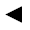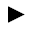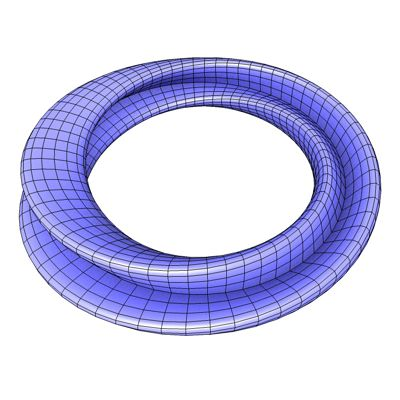[back]

## 3.56. Twisted Eight Torus

[next]The Twisted Eight Torus [ 16 ] is represented by the following equations. It is very similar to the 8-torus .

 x = (R+r (cos(u/2) sin(v)-sin(u/2) sin(2 v))) cos(u) 3-187 y = (R+r (cos(u/2) sin(v)-sin(u/2) sin(2 v))) sin(u) 3-188 z = r (sin(u/2) sin(v) + cos(u/2) sin(2v)) 3-189

The constants R and r determine the appearance of the figure.

To represent the area, the two parameters u and v can assume the following values ​​(domain of definition), for example.

 u is an element from the set of numbers [0, 2 pi] v is an element of the number set [0, 2 pi]

Since the rotated eight torus is a closed figure, the definition range must be adhered to exactly, it cannot be changed with the plugin.
The plugin creates an optimized mesh without duplicate points and unconnected polygons.Fig. 60

Fig. 60a shows the torus cut open in the middle. The torus cross section is twisted by 90°.Fig. 60a